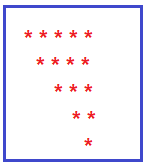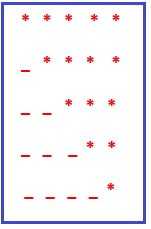# Downward Mirrored Right Triangle Star Pattern Program in C#

## Downward/Reversed Mirrored Right Triangle Star Pattern Program in C#

In this article, I am going to discuss How to implement the Downward/Reversed Mirrored Right Triangle Star Pattern Program in C# with Examples. Please read our previous article where we discussed Downward Triangle Star Pattern Program in C#. Please have a look at the below image which shows the Downward/Reversed Mirrored Right Triangle Star Pattern.##### Understanding the Downward Mirrored Right Triangle Star Pattern

If we want to create a downward mirrored right triangle star pattern with five rows, then

1. In the first row, we have 5 stars and 0 blank spaces in starting.
2. In the second row, we have 4 stars and 1 blank space in starting.
3. In the third row, we have 3 stars and 2 blank spaces in starting.
4. In the fourth row, we have 2 stars and 3 blank spaces in starting.
5. In the fifth row, we have 1 star and 4 blank spaces at the starting as shown in the below image.1. From the above pattern, it is clear that at each row number of stars is decreasing -1 at each row.
2. For example, the 1st row has 5 stars, the 2nd row has 4 stars and the 3rd row has 3 stars, and so on.
3. From the above pattern, it is clear that at each row number of blank spaces is increasing +1 at each row. For example, the 1st row has 0 space, the 2nd row has 1 space, the 3rd row has 2 spaces, and so on.
##### Calculate the number of stars in each row

Suppose the number of rows is 5 then

1. In the first row, we have 5(equal to row) stars.
2. In the second row, we have 4 (row-1) stars.
3. In the third row, we have 3 (row-2) stars.
4. In the fourth row, we have 2 (row -3) stars.
5. In the fifth row, we have 1 (row – 4) star.
##### How to implement the Downward Mirrored Triangle Star Pattern in C#?

To solve the above pattern,

1. We are going to use three for loops. One outer for loop and two inner for loops.
2. The outer for loop will be used to handle the rows one by one and the two inner for loops will be used to handle the columns one by one.
3. The first inner for loop will be used to calculate the number of blank spaces in each row.
4. The second inner for loop will be used to calculate the number of stars in each row.
5. The outer loop job is to go on a new line after printing the current row stars and blank spaces.
##### C# Program to Print Downward Mirrored Right Angle Triangle Star Pattern.

The following C# program will allow the user to input the number of rows and then print the downward mirrored right angle triangle star pattern on the console.

```using System;
public class DownwardMirroredTriangleStarPattern
{
public static void Main()
{
Console.WriteLine("Enter number of Rows :");

for (int i = 0; i < rows; i++)
{
int j = 0;
for (j = 0; j < i; j++)
{
Console.Write(" ");
}

/*
* rows-i
* first row (5-1) 0 to 4 = 5 stars
* second row (5-2) 0 to 3 = 4 stars
* third row (5-3) 0 to 2 = 3 stars
* and so on.
* */
for (j = 0; j < (rows - i); j++)
{
Console.Write("*");
}
Console.WriteLine();
}